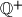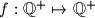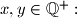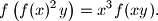### IMO Shortlist 2010 problem A5

Kvaliteta:
Avg: 2,7
Težina:
Avg: 8,0
Denote by$\mathbb{Q}^+$ the set of all positive rational numbers. Determine all functions$f : \mathbb{Q}^+ \mapsto \mathbb{Q}^+$ which satisfy the following equation for all$x, y \in \mathbb{Q}^+:$Proposed by Thomas Huber, Switzerland
Izvor: Međunarodna matematička olimpijada, shortlist 2010